• lda和神经网络
2021-05-17 17:12:44

## 前言

文中涉及代码及图片，可参见MATLAB基于PCA-LDA模糊神经网络的人脸识别，有需要的小伙伴可自行下载订阅，谢谢大家！

基于PCA-LDA和粗糙集模糊神经网络的人脸识别，一方面在应用前景上是十分广阔的，可以为IT行业创造更好的经济效益。

另一方面，它相对于其它的生物特征识别更加方便。

至于环境方面，人脸识别对环境并没有什么影响。

图像获取功能：该模块的功能是从图像库中获取图片，获取的图像必须能够在开发环境中可以显示，以便进行图像的处理和识别。

图像预处理功能：该模块的功能包括图像光线强弱的补偿，图像的灰度化处理，去噪，均衡化后的直方图，以此达到图像对比后增强的目的。

图像特征提取功能：改模块的功能是在处理后的图像进行训练，然后将训练后的图像的眼睛，鼻子，嘴巴等生物特征提取出来。

图像识别功能：该模块部分是将需要进行检测的图像的特征提取出来与数据库的图像的特征进行比较，以此达到人脸识别的功能。## 01 代码执行

先解压image-base压缩包与.m文件放在一起

然后在主窗口依次运行以下函数

saveORLimage.m将ORL人脸库分为测神经网络 人脸识别 图像处理 matlab 人工智能
更多相关内容
• MATLAB基于PCA-LDA模糊神经网络的人脸识别
• 基于Word2vec和LDA的卷积神经网络文本分类模型.pdf
• 图像识别5：LDA 与 SVM+神经网络+支持向量机 实验目的：熟练支持向量机的使用，掌握线性判别分析使用，以及 knn、k-means 聚类的使用方法。 实验内容：（1）LDA与SVM（2）KNN支持向量机（3）Kmean聚类

# 一、实验目的

熟练支持向量机的使用，掌握线性判别分析使用，以及 knn、k-means 聚类的使用方法。

# 二、 实验内容及结果

1、实验十一——线性判别分析 LDA 与支持向量机 SVM 的综合实验
（1） 根据多类人脸图，实现线性判别分析，求得投影矩阵 W；
（2） 将 W 作用在测试样本上，画出测试样本在投影后的分布图。（d’=2）；
（3） 根据投影后的训练数据和测试数据，使用支持向量机获得分类精度；
（4） 找出使得支持向量机分类精度最高的 d’。
2、实验十二——KNN 分类器与支持向量机的对比实验
（1） 选取合适的数据集，进行训练集和测试集的划分；
（2） 使用支持向量机进行分类，分析参数的影响；
（3） 使用 KNN 分类器进行分类，分析参数的影响；
（4） 对比两种分类器的最优分类精度。
3、实验十三——K-means 聚类算法实验
（1）使用 k-means 聚类完成三维高斯分布数据的分类，以及结果可视化；
（2）使用 k-means 聚类实现鸢尾花数据的分类。

## （1）LDA与SVM

读取和划分与实验十一样，因此直接读取数据集。
根据多类人脸图，实现LDA线性判别分析，求得投影矩阵 W。
结果可视化。这里首先取三原色随机数，然后通过eval函数调用不同的变量值。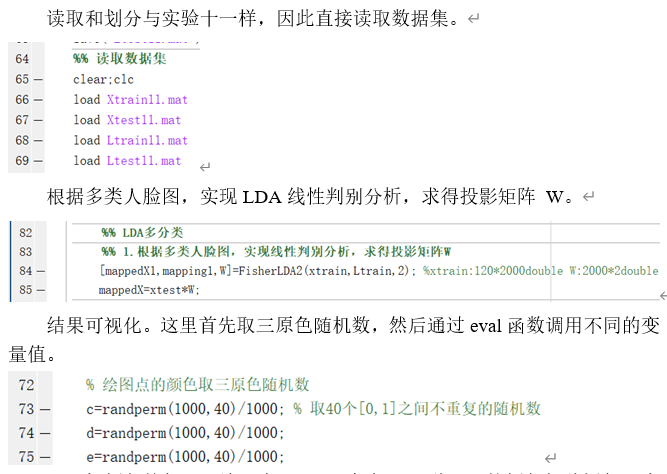取随机颜色的代码理论是生成3*40个点，40种不同的颜色每种颜色3个点，但由于’color’,[range,range,range]存在取到相同颜色的情况，因此看不出LDA降维后测试集的标签结果。尝试通过randperm函数强制让他取到不同的颜色。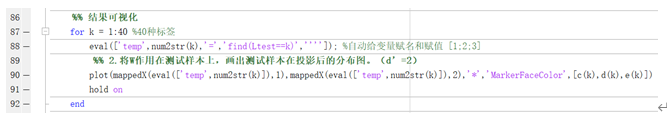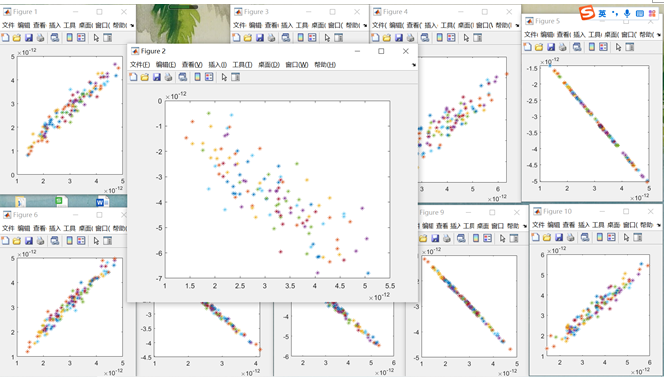可以看出，分类结果较好，颜色一样的基本都在一团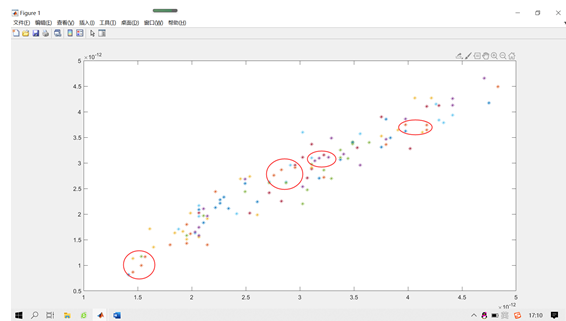>> plot(mappedX(temp1,1),mappedX(temp1,2),'*','MarkerFaceColor',[c(1),d(1),e(1)])
>> plot(mappedX(temp2,1),mappedX(temp2,2),'*','MarkerFaceColor',[c(1),d(1),e(1)])
>> plot(mappedX(temp3,1),mappedX(temp3,2),'*','MarkerFaceColor',[c(1),d(1),e(1)])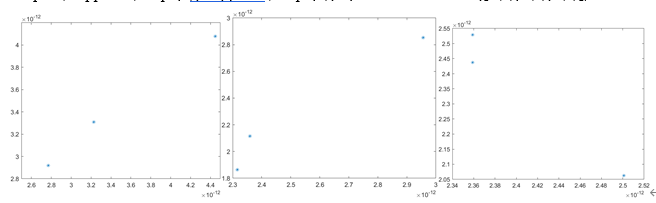读取数据集后对分类精度d’遍历，以找到最高的精度。
降维后导入支持向量机。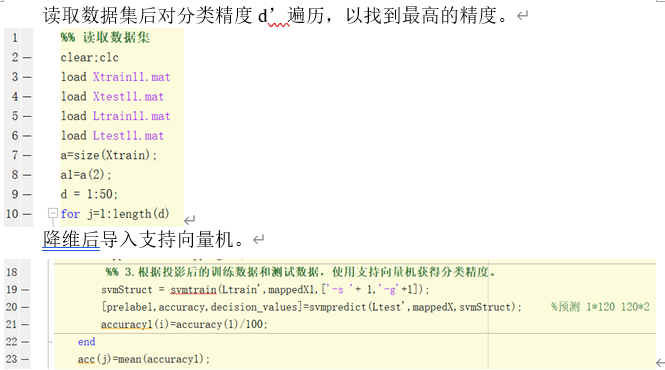## （2）KNN和支持向量机

选取的数据集为实验五的MIT室内场景数据集。使用支持向量机对其进行分类时，主要考虑参数’-s’svm类型和’-t’损失函数类型对分类精度的影响，对-s和-t参数循环取值为1到4。
可视化绘图。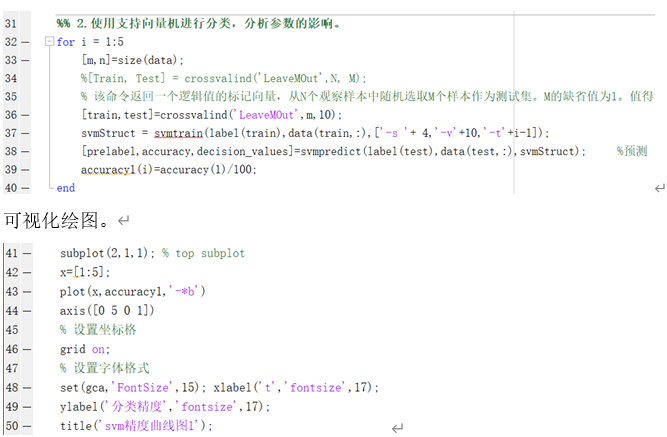使用 knn 对其进行分类，参数 k 取 1到10。
可视化绘图。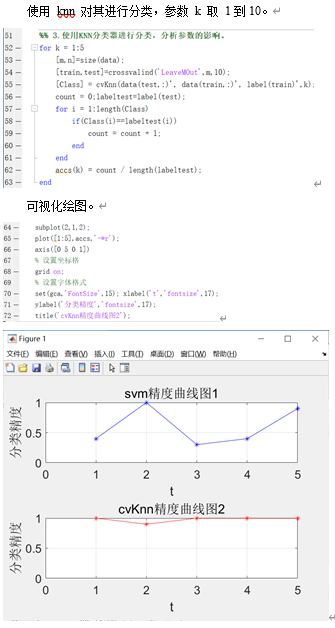## （3）Kmean聚类

使用高斯函数生成三维高斯的分布数据。
其他三类数据同理。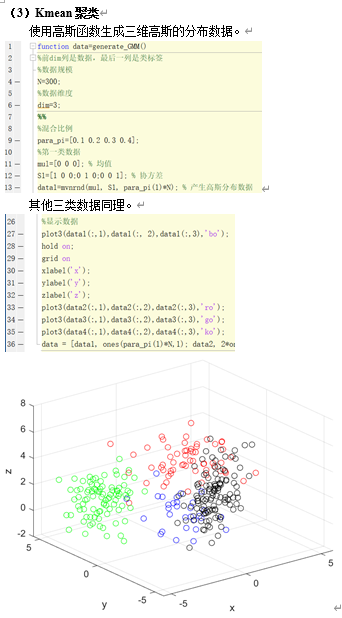生成三维高斯分布数据并可视化。
中心点可视化。
可视化。
原来可视化的代码如下：
改进：将一维矩阵的eval函数改进为二维矩阵，读写速度更快且更方便。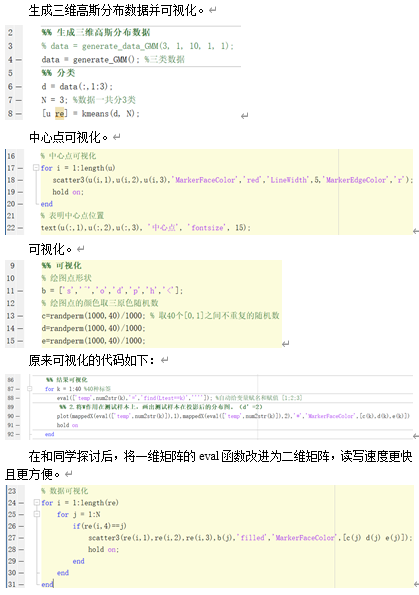将 iris 数据集导入后，使用 k-means 聚类对其进行分类。对于分类的每个类别，将其与原数据的类别进行对比，选取聚类类别个数的最多的为标准，将其作为这一项的分类类别。
准确率为88.666667%。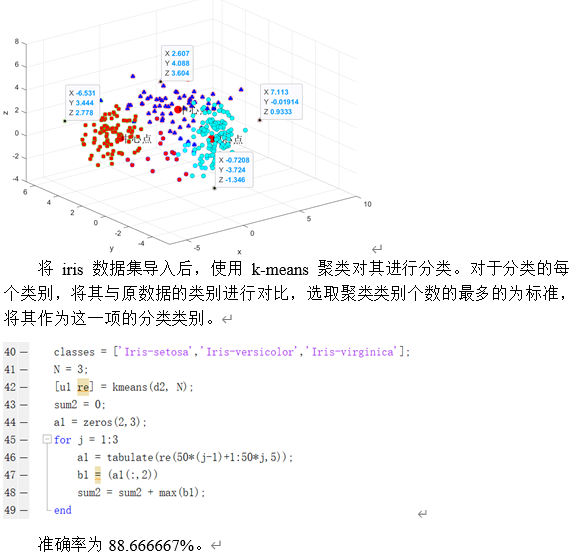# 三、实验心得

通过本次实验，熟练支持向量机的使用，掌握线性判别分析使用，以及 knn、k-means 聚类的使用方法。通过实验发现了学习率的知识盲点，通过查阅文献及课本及时进行了查缺补漏。对数学与编程的应用中有了更深的理解，和同学交流后，代码的时空复杂度降低，并且复用性得到了较大的提升。

# 四、实验源码

## 实验十一：线性判别分析 LDA 与支持向量机 SVM

% 1.根据多类人脸图，实现线性判别分析，求得投影矩阵W 。
% 2.将W作用在测试样本上，画出测试样本在投影后的分布图。（d’=2）
% 3.根据投影后的训练数据和测试数据，使用支持向量机获得分类精度。
% 4.找出使得支持向量机分类精度最高的d’。
%% 设置参数
clc;clear;close all;
warning('off') %关掉警告
base_path = 'D:\Desktop\大三上\神经网络\11\ORL人脸库';
path = string();
subpath = dir( base_path );
a=10; %留出法次数
%% 读取指定路径单文件夹下，all文件夹内的all图像
all_imgnum=0;all_e=0;all_f=0;
for i = 1:length(subpath)-2 % 读取单文件夹下，all文件夹% 1,2分别是.和..% 先获取第一个子文件路径，然后获取第二个子文件路径
sub_path = fullfile(base_path, subpath(i+2).name);% disp(sub_path); % D:\Desktop\大三上\神经网络\11\ORL实验\s12
image_name = dir([sub_path,'\*.bmp']); % 获取文件夹里的所有.bmp格式图像信息% disp(image_name(1).name); % 1.bmp
img_num=length(image_name); % 文件夹里图像个数% disp(img_num); %
all_imgnum=all_imgnum+img_num;% disp(all_imgnum); % 608 1013 1210 1872
e=round(img_num*2/3); % 留出法划分比例
f=img_num-e;
all_e=all_e+e;
all_f=all_f+f;
%% 获取图片数据
for j = 1:img_num % 获取子文件夹下图片的路径
% fullfile函数利用文件各部分信息创建并合成完整文件名
img_path = fullfile(sub_path, image_name(j).name); % 子文件夹+图片名称
end
image = double(imresize(read_img, [1,2000]));  % 图片统一大小，指定长宽[1,2000]
phi(all_imgnum-img_num+j,:)=image;  % 存放每个图片data于phi矩阵中，一行存放一个图像
end
end
%% 留出法a次二划分互斥的训练集和测试集 数据
for k=1:a
c=1;d=1;
for m=1:length(subpath)-2
% 划分训练集和测试集比例(2:1划分）
num=randperm(img_num); %打乱列序列
train_num=num(1:e); %取打乱序列的前60%
test_num=num(e+1:end); %取打乱序列的后40% %end直到数组结尾
% 划分数据
for ai=1:length(train_num)
phi0(c,:)=phi((m-1)*img_num+train_num(ai),:);
c=c+1;
end
for bi=1:length(test_num)
phi1(d,:)=phi((m-1)*img_num+test_num(bi),:);
d=d+1;
end
%% 存放图片训练集label于矩阵Ltrain % 定义标签，1234
Ltrain(1,c-length(train_num):c-1)=m*ones(1,length(train_num));% 行拼接每个标签给对应的图片个数拼成一行
Ltest(1,d-length(test_num):d-1)=m*ones(1,length(test_num));
end
Xtrain{1,k}=phi0;%第k次留出法的训练集
Xtest{1,k}=phi1;
end
%% 保存划分后的数据集
save('Xtrain11.mat','Xtrain'); % 存数据
save('Xtest11.mat','Xtest');
save('Ltrain11.mat','Ltrain');% 存标签
save('Ltest11.mat','Ltest');
%% 2.将W作用在测试样本上，画出测试样本在投影后的分布图。（d’=2）
%% 读取数据集
clear;clc
a=size(Xtrain);
a1=a(2);
% 绘图点的颜色取三原色随机数
c=randperm(1000,40)/1000; % 取40个[0,1]之间不重复的随机数
d=randperm(1000,40)/1000;
e=randperm(1000,40)/1000;
for i = 1:a1
% xtrain=Xtrain(1,i);
xtest=cell2mat(Xtest(1,i)); % 元胞数组转化为矩阵
xtrain=cell2mat(Xtrain(1,i));
b=size(xtest);
figure(i)
%% LDA多分类
[mappedX1,mapping1,W]=FisherLDA2(xtrain,Ltrain,2); %xtrain:120*2000double W:2000*2double
mappedX=xtest*W;
%% 结果可视化
for k = 1:40 %40种标签
eval(['temp',num2str(k),'=','find(Ltest==k)','''']); %自动给变量赋名和赋值 [1;2;3]
plot(mappedX(eval(['temp',num2str(k)]),1),mappedX(eval(['temp',num2str(k)]),2),'*','MarkerFaceColor',[c(k),d(k),e(k)])
hold on
end
end
%% 4.找出使得支持向量机分类精度最高的d’。
% 读取数据集
clear;clc
a=size(Xtrain);
a1=a(2);
d = 1:50;
for j=1:length(d)
for i = 1:a1
% xtrain=Xtrain(1,i);
xtest=cell2mat(Xtest(1,i)); % 元胞数组转化为矩阵
xtrain=cell2mat(Xtrain(1,i));
%% LDA多分类
[mappedX1,mapping1]=lda222(xtrain,Ltrain,d(j)); %xtrain:120*2000double W:2000*2double
mappedX=xtest*mapping1.M;
%% 3.根据投影后的训练数据和测试数据，使用支持向量机获得分类精度。
svmStruct = svmtrain(Ltrain',mappedX1,['-s '+ 1,'-g'+1]);
[prelabel,accuracy,decision_values]=svmpredict(Ltest',mappedX,svmStruct);     %预测 1*120 120*2
accuracy1(i)=accuracy(1)/100;
end
acc(j)=mean(accuracy1);
end
disp(acc);
plot(d,acc,'-*b')
axis([0 50 0 1]) % 设置坐标格
grid on;
set(gca,'FontSize',15); xlabel('t','fontsize',17); % 设置字体格式
ylabel('分类精度','fontsize',17);
title('精度曲线图');


## 实验十二：支持向量机与KNN

%% 1.选取合适的数据集，进行训练集和测试集的划分。
% 根据两类人脸图，实现二分类实验
clc;clear;close all;
warning('off') %关掉警告
base_path = 'D:\Desktop\大三上\机器学习\10\人脸图';
path = string();
subpath = dir( base_path );
%% 训练阶段
%读取样本并计算hog特征
all_imgnum=0;
for i = 1:length(subpath)-2 % 读取单文件夹下，all文件夹% 1,2分别是.和..% 先获取第一个子文件路径，然后获取第二个子文件路径
sub_path = fullfile(base_path, subpath(i+2).name);% disp(sub_path); % D:\Desktop\大三上\神经网络\数据\MIT室内场景\airport_inside
image_name = dir(sub_path); % 获取文件夹里的所有图像信息% disp(image_name(3).name); % airport_inside_0001.jpg
img_num=length(image_name)-2; % 文件夹里图像个数% disp(img_num); % 608 405 197 662
all_imgnum=all_imgnum+img_num;% disp(all_imgnum); % 608 1013 1210 1872
%% 获取图片数据
for j = 1:img_num % 获取子文件夹下图片的路径
% fullfile函数利用文件各部分信息创建并合成完整文件名
img_path = fullfile(sub_path, image_name(j+2).name); % 子文件夹+图片名称
image = imresize(read_img, [64,64]);  % 图片统一大小，指定长宽[64,64]
end
hog =hogcalculator(image);
data(all_imgnum-img_num+j,1:1764)=hog;
end
% 存放图片label  % 定义标签，0:负样本 1:正样本
label(all_imgnum-img_num+1:all_imgnum,1)=(i-1)*ones(img_num,1);
end
%% 2.使用支持向量机进行分类，分析参数的影响。
for i = 1:5
[m,n]=size(data);
%[Train, Test] = crossvalind('LeaveMOut',N, M);
% 该命令返回一个逻辑值的标记向量，从N个观察样本中随机选取M个样本作为测试集。M的缺省值为1。值得注意的是，LeaveMOut在循环中使用不能保证产生的是互补集合，即每次循环的随机选取是独立的。如果要用互补的话还是使用Kfold命令。
[train,test]=crossvalind('LeaveMOut',m,10);
svmStruct = svmtrain(label(train),data(train,:),['-s '+ 4,'-v'+10,'-t'+i-1]);
[prelabel,accuracy,decision_values]=svmpredict(label(test),data(test,:),svmStruct);     %预测
accuracy1(i)=accuracy(1)/100;
end
subplot(2,1,1); % top subplot
x=[1:5];
plot(x,accuracy1,'-*b')
axis([0 5 0 1])
% 设置坐标格
grid on;
% 设置字体格式
set(gca,'FontSize',15); xlabel('t','fontsize',17);
ylabel('分类精度','fontsize',17);
title('svm精度曲线图1');
%% 3.使用KNN分类器进行分类，分析参数的影响。
for k = 1:5
[m,n]=size(data);
[train,test]=crossvalind('LeaveMOut',m,10);
[Class] = cvKnn(data(test,:)', data(train,:)', label(train)',k);
count = 0;labeltest=label(test);
for i = 1:length(Class)
if(Class(i)==labeltest(i))
count = count + 1;
end
end
accs(k) = count / length(labeltest);
end
subplot(2,1,2);
plot([1:5],accs,'-*r');
axis([0 5 0 1])
% 设置坐标格
grid on;
% 设置字体格式
set(gca,'FontSize',15); xlabel('t','fontsize',17);
ylabel('分类精度','fontsize',17);
title('cvKnn精度曲线图2');


## 实验十三：k-means聚类

%% 1、使用k-means聚类完成三维高斯分布数据的分类，以及结果可视化；
%% 生成三维高斯分布数据
% data = generate_data_GMM(3, 1, 10, 1, 1);
data = generate_GMM(); %三类数据
%% 分类
d = data(:,1:3);
N = 3; %数据一共分3类
[u re] = kmeans(d, N);
%% 可视化
% 绘图点形状
b = ['s','^','o','d','p','h','<'];
% 绘图点的颜色取三原色随机数
c=randperm(1000,40)/1000; % 取40个[0,1]之间不重复的随机数
d=randperm(1000,40)/1000;
e=randperm(1000,40)/1000;
% 中心点可视化
for i = 1:length(u)
scatter3(u(i,1),u(i,2),u(i,3),'MarkerFaceColor','red','LineWidth',5,'MarkerEdgeColor','r');
hold on;
end
% 表明中心点位置
text(u(:,1),u(:,2),u(:,3), '中心点', 'fontsize', 15);
% 数据可视化
for i = 1:length(re)
for j = 1:N
if(re(i,4)==j)
scatter3(re(i,1),re(i,2),re(i,3),b(j),'filled','MarkerFaceColor',[c(j) d(j) e(j)]);
hold on;
end
end
end
%% 2、使用k-means聚类实现鸢尾花数据的分类；
clear;clc
d2 = iris(:,1:4);
iris(1:50,5)=1;
iris(51:100,5)=2;
iris(101:150,5)=3;
l = iris(:,5);
classes = ['Iris-setosa','Iris-versicolor','Iris-virginica'];
N = 3;
[u1 re] = kmeans(d2, N);
sum2 = 0;
a1 = zeros(2,3);
for j = 1:3
a1 = tabulate(re(50*(j-1)+1:50*j,5));
b1 = (a1(:,2))
sum2 = sum2 + max(b1);
end
fprintf("准确率为%f%%",sum2/150*100);

展开全文支持向量机 神经网络 机器学习
• 本篇文章介绍一下机器学习中最为常见的数据降维技术PCA和LDA，随着深度学神经网络的发展PCA和LDA的应用几乎越来越少了，尤其是LDA，但是这两种降维的思想还是值得我们学习的。 PCA 主成分分析（PCA）是一种无监督...

本篇文章介绍一下机器学习中最为常见的数据降维技术PCA和LDA，随着深度学神经网络的发展PCA和LDA的应用几乎越来越少了，尤其是LDA，但是这两种降维的思想还是值得我们学习的。

## PCA

主成分分析（PCA）是一种无监督学习的降维方法，其主要思想非常非常简单，即寻找一组正交基对原始数据进行线性变换，使变换后的数据在某一个正交基方向上方差最大，从而尽最大可能地将原始数据投影到该方向上，如下图。那么，我们如何找到这组正交基呢？

别急，在寻找正交基之前，我们还需要对数据进行中心化预处理，将所有数据搬移到坐标原点。然后假设在N维空间中的一个单位方向向量为w，我们只需要让所有数据样本在w上的投影方差最大即可。上式中中间的一大坨不就是协方差矩阵嘛！不过别忘了，我们还有一个约束条件，w的模是等于1的哦，所有优化问题变为如下：好了，到了我们最拿手的拉格朗日乘子法了，我们把约束问题转为无约束优化问题，即：呵呵，就这？
我们发现原来方差的最大值居然是协方差矩阵的最大特征值，那这个事就变简单了，我们只需要先求出协方差矩阵的所有特征值，然后对特征值进行降序排序，最后选出前K（假设我们想将数据降到K维）大的特征值所对应的特征向量即可。但是有的人可能会问，特征向量一定是正交的吗？别忘了，我们的协方差矩阵可是实对称矩阵，学过线性代数的都知道实对称阵是一定可以对角化并存在一组正交基。我们只需计算数据在前K大的特征值所对应的特征向量上的投影这个事情就搞定了。

嗯，PCA就完事了。

## LDA

哦豁？LDA是啥？PCA不是已经可以降维了吗？
别忘了PCA是一种无监督降维方法，而LDA是一种有监督的降维方法，PCA只能通过数据之间的内在关系进行降维，而LDA则可以尽可能的利用标签的信息对数据进行降维。

线性判别分析（LDA）的思想也很简单，即数据的类间距离最大化，类内距离最小化，说白了，LDA就是想将同类数据紧凑的抱团。

首先根据标签，我们先计算每一类数据各个特征维度的中心，以二分类为例：然后计算其投影后的类间距离，其中然后计算类内方差：我们希望的目标是，类间方差最大，类内方差最小，因此优化目标可以写为：令类间散度矩阵为：类内散度矩阵为：因此优化目标重新写为对w求偏导，并令其等于0即可。于是得出，令，整理得,到这里我们发现，目标函数的最大值即为矩阵的最大特征值，与PCA类似，我们只需要找出前K大的特征值所对应的特征向量即为我们所求的正交变换矩阵。

展开全文机器学习
• 基于csp特征，然后实现LDA和bp神经网络的分类技术研究 Csp-lda，csp-bp的脑电波分类技术研究matlab
• 人工智能-基于Corr-LDA模型及卷积神经网络的图像理解方法.pdf
• 基于小波包-LDA神经网络的模拟电路故障诊断研究，肖远鹏，李志华，模拟电路故障诊断对于电子设备及系统的正常运行具有十分重要的意义。本文对模拟电路提出了一种改进的基于小波包-LDA预处理神经网��
• legend('SOFTmax预测值','真实值')xlabel('样本')title(['准确率=',num2str(Accuracy)]) 3 仿真结果 4 参考文献 博主简介：擅长智能优化算法、神经网络预测、信号处理、元胞自动机、图像处理、路径规划、无人机等...

## 1 简介

本文以气体传感器收集的数据来训练几种不同的分类器，并测试了正确率

这里仅演示了最基本的使用方法，各个参数的设定还是参考帮助文档为好。

数据来源：http://archive.ics.uci.edu/ml/datasets/Gas+Sensor+Array+Drift+Dataset

数据分为6类气体，由不同的时间段采集，并由时间段分成了10个数据集，各个数据集的详细情况可以见网站的说明。总之，这里有6类气体的数据，不过需要注意的时，由于传感器材质或工艺水平的原因，随着时间的变化，传感器本身会产生漂移。因此，如果用第10个batch上的数据来训练一个分类器，用第1个batch上面的数据来进行测试，正确率可能会很低。这也是现在需要解决的问题。

训练数据600个，测试数据3000个，

固定初始参数时，各个分类器的正确率如下：

Bayes: 60%

BP: 90.5%

Elman: 77.5%

KNN: 97.5333%

LDA: 84.4667%

RBF: 97.7%

Softmax: 98.1667%

## 2 部分代码

% /* %  * Expanse@yeah.net 2018/07/27%  * 每一列表示一个样本%  */​clear; clc;load Batch.mat C_data C_label;  % 加载数据​Data = C_data{10};              % 数据Label = C_label{10};            % 标签​indices = crossvalind('Kfold',3600,6);      % 随机trainindices = (indices == 1);        testindices = ~trainindices;  traindata = Data(:, trainindices);    testdata = Data(:, testindices);    % 训练数据600个trainlabel = Label(:, trainindices);  testlabel = Label(:, testindices);  % 测试数据3000个​% 数据归一化（可选）[traindata,PS]=mapminmax(traindata,0,1);              %要进行一次转置，这样的归一化是对列（也就是每个传感器响应曲线）的归一化，也有的是对每一次采样得到的数据进行归一化。 testdata=mapminmax('apply',testdata,PS);​setdemorandstream(pi);        % 固定随机值​Softmaxnet = trainSoftmaxLayer(traindata,trainlabel,'MaxEpoch',500);​Y = Softmaxnet(testdata);    % 统计分类正确率figureplotconfusion(testlabel,Y);testClassPrediction=compet(Y);testClass=vec2ind(testClassPrediction);ttest=vec2ind(testlabel);​count = sum(testClass == ttest);​Accuracy = 100*count/length(testlabel);disp('the Accuracy is ');disp(Accuracy);figureplot(testClass ,'ro-');hold onplot(ttest ,'b*-');legend('SOFTmax预测值','真实值')xlabel('样本')title(['准确率=',num2str(Accuracy)])

## 3 仿真结果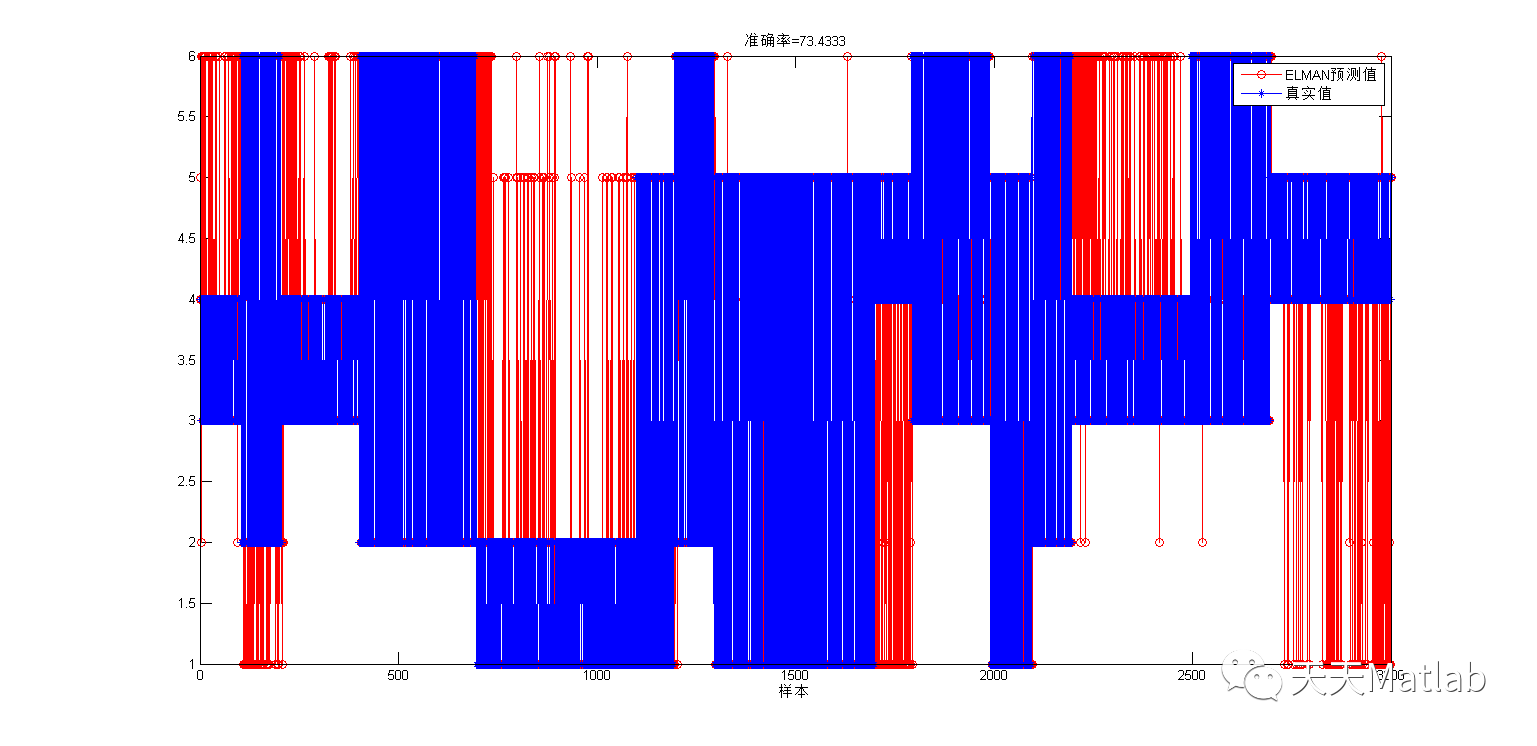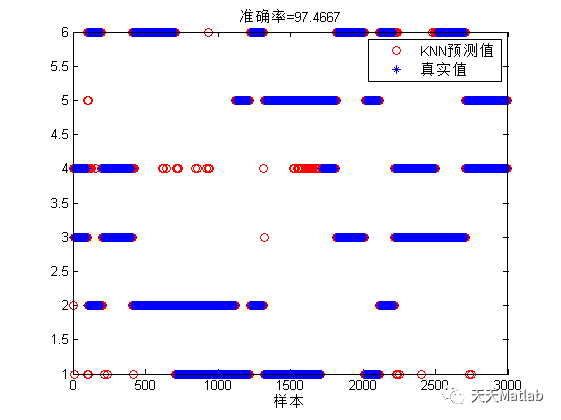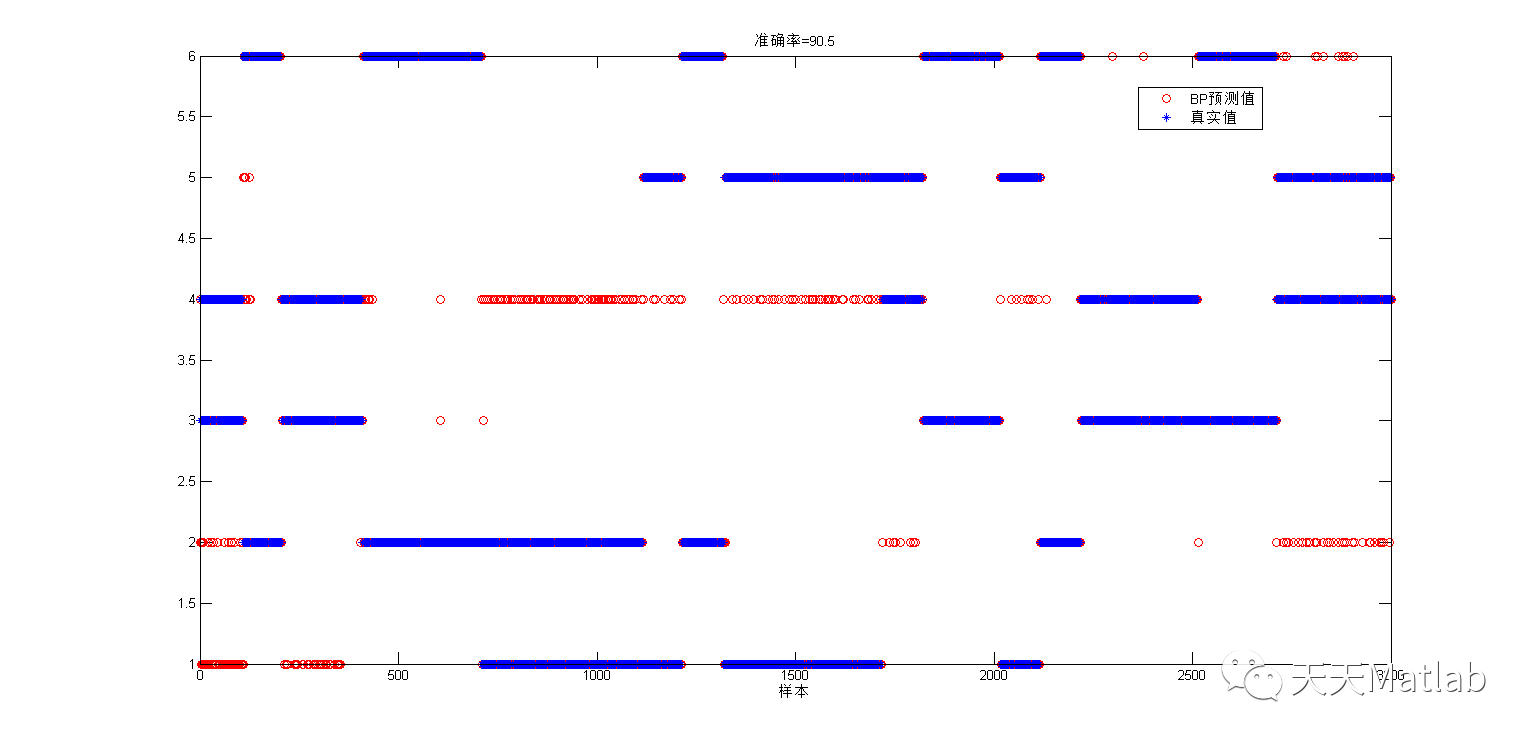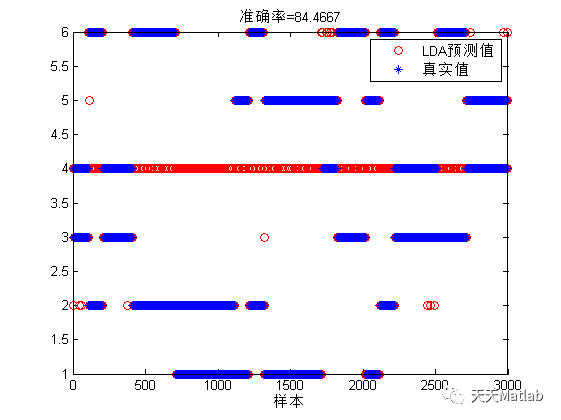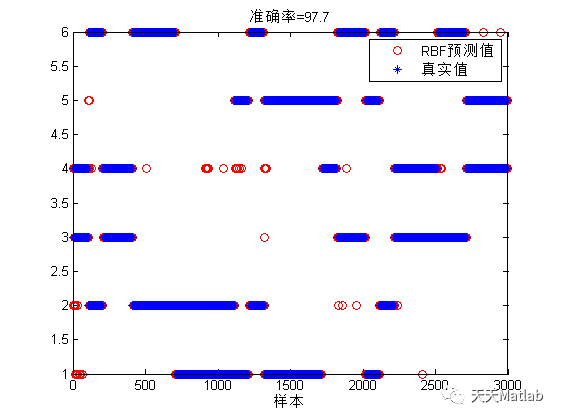## 4 参考文献

### 博主简介：擅长智能优化算法、神经网络预测、信号处理、元胞自动机、图像处理、路径规划、无人机等多种领域的Matlab仿真，相关matlab代码问题可私信交流。

部分理论引用网络文献，若有侵权联系博主删除。

展开全文神经网络 分类 matlab
• 融合PCA、LDA和SVM算法的人脸识别.pdf
• 针对人体行为识别问题，提出一种基于径向基函数（BP）神经网络的人体行为分类算法。首先，利用奇异值分解（SVD）算法提取视频每一帧的奇异值，将每一帧的奇异值按照行拼接起来即为一个视频的样本，样本按照行排成...人体行为识别 PCA LDA BP神经网络
• 基于pca lda 粗糙集 模糊神经网络的人脸识别程序 在matlab上可直接运行matlab
• 基于PCA-LDA与蚁群优化BP神经网络的人脸识别算法.pdf
• 除机器学习安装工具包外，里面还有包含了多种机器学习算法里，如BP神经网络、SVM、SOM等。希望可以帮助到大家
• 基于改进卷积神经网络与集成学习的人脸识别算法.pdf
• 使用matlab训练基本的神经网络，数据是使用的6类气体的数据，共有3600个，分别测试了7个分类器的性能
• 基于神经网络的语音情感分析python实现，内部函数聚集以及详细教程
• 在这里，我训练了支持向量机，线性判别分析四层前馈神经网络，以对来自CIFAR-10数据集的10个图像类别进行分类，从而以62.7％的SVM实现了最高的准确性。 该实验的关键问题是发现用于降低尺寸的PCA和LDA的非传统组合...
• pca+lda+粗糙集+模糊神经网络 saveORLimage.m将ORL人脸库分为测试集ptest训练集pstudy存为imagedata.mat 1.savelda.m将人脸库先进行pca降维，再用lda进行特征提取，得到新的测试集ldatest训练集ldastudy存为...
• 从零开始的ML算法 包括Softmax回归，神经网络（常规），KNN，LDA
• pca+lda+粗糙集+模糊神经网络 saveORLimage.m将ORL人脸库分为测试集ptest训练集pstudy存为imagedata.mat 1.savelda.m将人脸库先进行pca降维，再用lda进行特征提取，得到新的测试集ldatest训练集ldastudy存为...
• Deep Feature Extraction and Classification of Hyp全文翻译（带公式）
•神经网络 深度学习 人工智能
• 近年来提出的神经主题模型变体的PyTorch实现包括NVDM-GSM，WTM-MMD（W-LDA），WTM-GMM，ETM，BATMGMNTM。 该项目的目的是为神经主题模型提供一个实用且可行的示例，以促进相关领域的研究。 模型的配置与论文中...
• 基于PCA_LDA和协同表示分类的人脸识别算法.pdf...